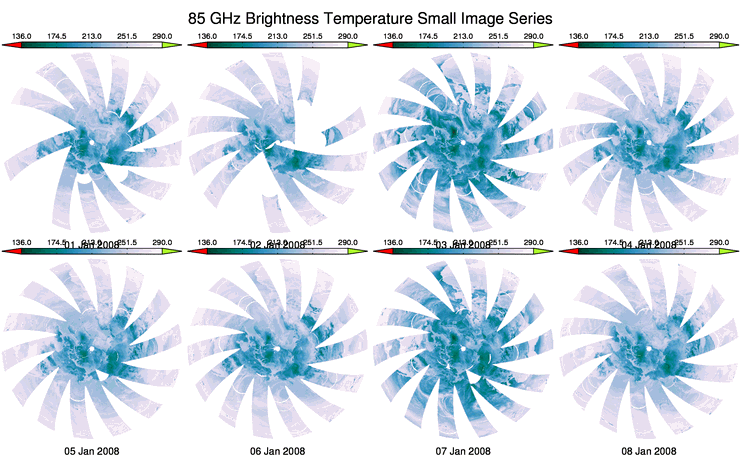# Leaving Room Around Images

QUESTION: I love cgImage for displaying images. And I especially like it that I can display multiple images in a window by using !P.Multi to determine the window position for the image. But I have a problem. I often want to associate a color bar or other image annotation with the image and the window position created by !P.Multi doesn't leave me enough room to do that. Is it possible to adjust the !P.Multi window position so I have a little more room for these kinds of flourishes? Here is an example of what my output looks like when I try to add both a color bar and a date for the image.An example of how the image annotations overlap in the middle of the page.ANSWER: Yes, indeed. You can use the MultiMargin keyword to cgImage to adjust the image position calculated by !P.Multi to leave room for the color bar and the other annotations you wish to use. MultiMargin is a four-element array that allows you to specify additional margins for the bottom, left side, top, and right side of the image, respectively. To leave room for both the color bar and the data annotation in your example, you might set MultiMargin=[4, 0, 4, 0]. Here is the code I modified from your example.

```    !P.Multi = [0,4,2]
!Y.OMargin = [2, 6]    FOR j=0,7 DO BEGIN
; Display the image. Return the displayed position in "p".
cgImage, scaledImage[j], /KEEP_ASPECT, POSITION=p, MULTIMARGIN=[4,0,4,0]
; Draw a colorbar above the image.
unit = !D.Y_CH_SIZE / Float(!D.Y_SIZE)       ydistance = 2.0 * unit
xdistance = 3.0 * !D.X_CH_SIZE / Float(!D.X_Size)
TVLCT, cgColor(self.oob_low_color, /TRIPLE), 253
TVLCT, cgColor(self.oob_high_color, /TRIPLE), 254
TVLCT, cgColor('white', /TRIPLE), 255
cgColorbar, NCOLORS=253, RANGE=[sclmin, sclmax], DIVISIONS=4, FORMAT='(F0.1)', \$
POSITION=[p+xdistance, p+ 0.01, p-xdistance, p+ydistance/2.], /TOP, \$
ANNOTATECOLOR=self.axes_color, XCharsize = 1.0, FONT=0, XTINKLEN=1.0, XMINOR=0
POLYFILL, [p+xdistance, p, p+xdistance, p+xdistance], \$
[p + 0.01,((p+ydistance/2.)-(p+ 0.01))/2. + (p+ 0.01), \$
p+ydistance/2.0, p+ 0.01], /NORMAL, \$
COLOR=cgColor(self.oob_low_color)
PLOTS, [p+xdistance, p, p+xdistance, p+xdistance], \$
[p + 0.01,((p+ydistance/2.)-(p+ 0.01))/2. + (p+ 0.01), \$
p+ydistance/2.0, p+ 0.01], /NORMAL, \$
COLOR=cgColor(self.axes_color)
POLYFILL, [p-xdistance, p, p-xdistance, p-xdistance], \$
[p + 0.01,((p+ydistance/2.)-(p+ 0.01))/2. + (p+ 0.01), \$
p+ydistance/2.0, p+ 0.01], /NORMAL, \$
COLOR=cgColor(self.oob_high_color)
PLOTS, [p-xdistance, p, p-xdistance, p-xdistance], \$
[p + 0.01,((p+ydistance/2.)-(p+ 0.01))/2. + (p+ 0.01), \$
p+ydistance/2.0, p+ 0.01], /NORMAL, \$
COLOR=FSC_Color(self.axes_color)
; Put a title under the image.
XYOUTS, (p - p) / 2 + p, /NORMAL, p - (1.5 * unit), timeStr[j], \$
ALIGNMENT=0.5, COLOR=cgColor(self.axes_color), CHARSIZE=0.8, FONT=0
; Make sure the position is undefined.       Undefine, p     ENDFOR
XYOUTS, 0.5, 0.95, /NORMAL, ALIGNMENT=0.5, CHARSIZE=1.25, FONT=0, plot_title
!Y.OMargin = [0,0]    !P.Multi = 0 ```

You can see the results in the figure below.Here the image position has been adjusted with additional margins for the annotations.